### Java程序设计语言

java 是一种可以撰写跨平台应用软件的面向对象的程序设计语言，是由Sun Microsystems公司于1995年5月推出的Java程序设计语言和Java平台（即JavaEE(j2ee), JavaME(j2me), JavaSE(j2se)）的总称。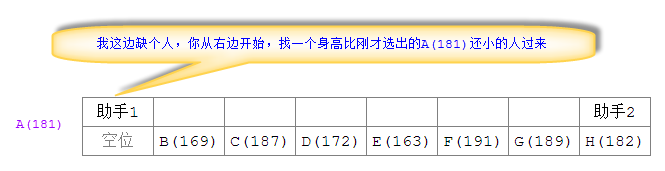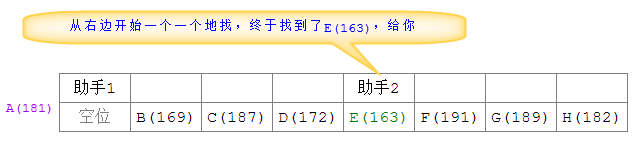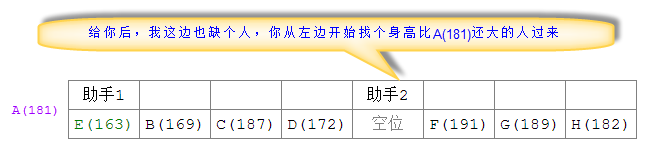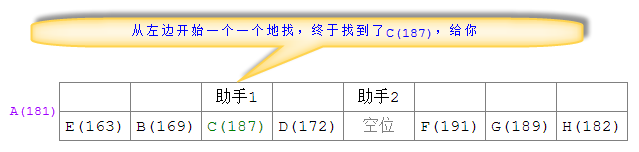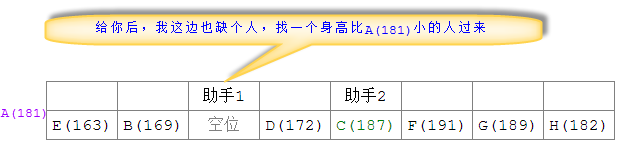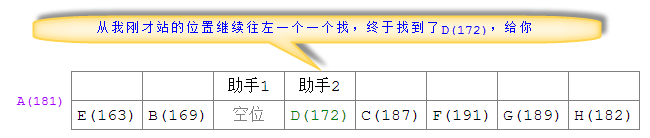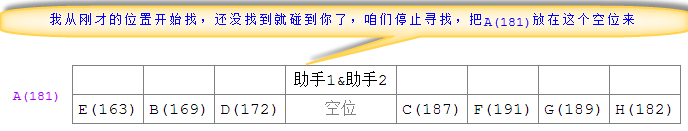``````
/**
* 对指定的数组中索引从start到end之间的元素进行快速排序
*
* @param array 指定的数组
* @param start 需要快速排序的数组索引起点
* @param end 需要快速排序的数组索引终点
*/
public static final void quickSort(int[] array, int start, int end) {
// i相当于助手1的位置，j相当于助手2的位置
int i = start, j = end;
int pivot = array[i]; // 取第1个元素为基准元素
int emptyIndex = i; // 表示空位的位置索引，默认为被取出的基准元素的位置
// 如果需要排序的元素个数不止1个，就进入快速排序(只要i和j不同，就表明至少有2个数组元素需要排序)
while (i < j) {
// 助手2开始从右向左一个个地查找小于基准元素的元素
while (i < j && pivot <= array[j])
j--;
if (i < j) {
// 如果助手2在遇到助手1之前就找到了对应的元素，就将该元素给助手1的"空位"，j成了空位
array[emptyIndex] = array[emptyIndex = j];
}

// 助手1开始从左向右一个个地查找大于基准元素的元素
while (i < j && array[i] <= pivot)
i++;
if (i < j) {
// 如果助手1在遇到助手2之前就找到了对应的元素，就将该元素给助手2的"空位"，i成了空位
array[emptyIndex] = array[emptyIndex = i];
}
}
// 助手1和助手2相遇后会停止循环，将最初取出的基准值给最后的空位
array[emptyIndex] = pivot;

// =====本轮快速排序完成=====

// 如果分割点i左侧有2个以上的元素，递归调用继续对其进行快速排序
if (i - start > 1) {
quickSort(array, 0, i - 1);
}
// 如果分割点j右侧有2个以上的元素，递归调用继续对其进行快速排序
if (end - j > 1) {
quickSort(array, j + 1, end);
}
}

//主方法
public static void main(String[] args) {
// =====使用快速排序法对表示8名运动员身高的数组heights进行从低到高的排序=====
// A(181)、B(169)、C(187)、D(172)、E(163)、F(191)、G(189)、H(182)
int[] heights = { 181, 169, 187, 172, 163, 191, 189, 182 };
// 调用快速排序方法
quickSort(heights, 0, heights.length - 1);
// 输出排序后的结果
for (int height : heights) {
System.out.println(height);
}
}

``````

``````
163
169
172
181
182
187
189
191
``````

``````
int partition(int[] a, int lo, int hi) {
int i = lo - 1, j = lo;
int v = a[hi];
while (j < hi) {
if (a[j] <= v) {
swap(a, ++i, j);
}
j++;
}
swap(a, i + 1, hi);
return i + 1;
}
``````

Java 中实现随机无重复数字的方法
Java编程中快速排序算法的实现及相关算法优化

java 合并排序算法、冒泡排序算法、选择排序算法、插入排序算法、快速排序算法的描述
Java中实现文件上传下载的三种解决方案(推荐)

JAVA中IP和整数相互转化的方法
java中out.print和out.write的方法